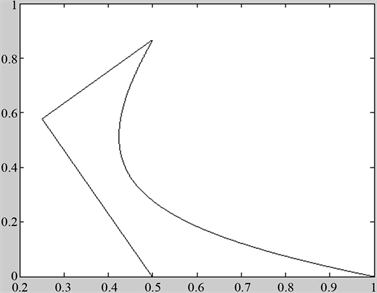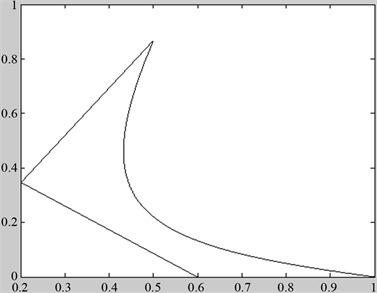﻿ 基于DP曲线构建PH样条

# 基于DP曲线构建PH样条Construction of PH Splines Based on DP Curves

Abstract: The PH curve is a special kind of polynomial parameter curve. The most significant advantage is that the arc length function is a polynomial. And the equidistant line can be represented by a rational polynomial curve, which is compatible with the CAD system. Based on the DP curve, a necessary and sufficient condition for a cubic plane DP curve to be a PH curve is obtained. The geometrical characteristics of the edge length and the angle of the control polygon are obtained and the definition of the DP-PH curve is obtained. The geometric feature condition of polygon is controlled by DP-PH. We describe the construction of a control polygon for a cubic DP-PH curve from geometric construction method, based on the procedure for a DP curve. Then the errors between DP curve and DP-PH curve are analyzed.

1. 引言

2. 三次DP-PH曲线的构造

2.1. 三次DP曲线

$P\left(t\right)=\underset{i}{\overset{3}{\sum }}{p}_{i}{D}_{i,3}\left(t\right)$

$\left[{D}_{0,3}\left(t\right),{D}_{1,3}\left(t\right),{D}_{2,3}\left(t\right),{D}_{3,3}\left(t\right)\right]=\left[{\left(1-t\right)}^{3},t\left(1-t\right)\left(2-t\right),t\left(1-t\right)\left(1+t\right),{t}^{3}\right]$

2.2. PH曲线

${x}^{\prime }\left(t\right)=w\left(t\right)\left({u}^{2}\left(t\right)-{v}^{2}\left(t\right)\right),{y}^{\prime }\left(t\right)=2w\left(t\right)u\left(t\right)v\left(t\right)$

2.3. DP-PH曲线几何特征条件

$a\left(t\right)={a}_{0}{D}_{0}^{1}\left(t\right)+a{D}_{1}^{1}\left(t\right)$

$b\left(t\right)={b}_{0}{D}_{0}^{1}\left(t\right)+b{D}_{1}^{1}\left(t\right)$

$a{\left(t\right)}^{2}-b{\left(t\right)}^{2}=2\left({a}_{0}^{2}-{b}_{0}^{2}\right){D}_{0}^{2}\left(t\right)+3\left({a}_{0}{a}_{1}-{b}_{0}{b}_{1}\right){D}_{1}^{2}\left(t\right)+2\left({a}_{1}^{2}-{b}_{1}^{2}\right){D}_{2}^{2}\left(t\right)$

$2a\left(t\right)b\left(t\right)=4{a}_{0}{b}_{0}{D}_{0}^{2}\left(t\right)+3\left({a}_{0}{b}_{1}+{a}_{1}{b}_{0}\right){D}_{1}^{2}\left(t\right)+4{a}_{1}{b}_{1}{D}_{2}^{2}\left(t\right)$

$x\left(t\right)={\int }_{0}^{t}a{\left(t\right)}^{2}-b{\left(t\right)}^{2}\text{d}t=\underset{k=0}{\overset{3}{\sum }}{x}_{k}{D}_{k}^{3}\left(t\right)$

$y\left(t\right)={\int }_{0}^{t}2a\left(t\right)b\left(t\right)\text{d}t=\underset{k=0}{\overset{3}{\sum }}{y}_{k}{D}_{k}^{3}\left(t\right)$

$\left\{\begin{array}{l}{p}_{1}={p}_{0}+\frac{1}{3}\left(2\left({a}_{0}^{2}-{b}_{0}^{2}\right),4{a}_{0}{b}_{0}\right)\\ {p}_{2}={p}_{1}+\frac{1}{3}\left(3\left({a}_{0}{a}_{1}-{b}_{0}{b}_{1}\right),3\left({a}_{0}{a}_{1}+{b}_{0}{b}_{1}\right)\right)\\ {p}_{3}={p}_{2}+\frac{1}{3}\left(2\left({a}_{1}^{2}-{b}_{1}^{2}\right),4{a}_{1}{b}_{1}\right)\end{array}$

$2L=3\sqrt{{L}_{1}{L}_{3}}$${\theta }_{1}={\theta }_{2}$

$q\left(t\right)$ 就是PH-DP曲线。

$q\left(t\right)$ 满足PH曲线的条件公式(2.1)，可以得到：

$\left\{\begin{array}{l}{d}_{01}=\frac{2\left({a}_{0}^{2}+{b}_{0}^{2}\right)}{3}\\ {d}_{12}=\sqrt{2\left({a}_{0}^{2}{a}_{1}^{2}+{b}_{0}^{2}{b}_{1}^{2}\right)}\\ {d}_{23}=\frac{2\left({a}_{1}^{2}+{b}_{1}^{2}\right)}{3}\end{array}$Figure 1. The geometric form of the cubic DP-PH curve

$\mathrm{cos}{\theta }_{1}=\frac{{d}_{01}^{2}+{d}_{12}^{2}-{d}_{02}^{2}}{2{d}_{12}{d}_{23}}$

$\mathrm{cos}{\theta }_{2}=\frac{{d}_{12}^{2}+{d}_{23}^{2}-{d}_{13}^{2}}{2{d}_{12}{d}_{23}}$

${d}_{02}^{2}=\frac{4}{9}\left({a}_{0}^{2}+{b}_{0}^{2}\right)\left[9{\left({a}_{0}+{a}_{1}\right)}^{2}+9{\left({b}_{0}+{b}_{1}\right)}^{2}\right]$

${d}_{13}^{2}=\frac{4}{9}\left({a}_{1}^{2}+{b}_{1}^{2}\right)\left[9{\left({a}_{0}+{a}_{1}\right)}^{2}+9{\left({b}_{0}+{b}_{1}\right)}^{2}\right]$

$\mathrm{cos}{\theta }_{1}=\mathrm{cos}{\theta }_{2}=\frac{-4\left({a}_{0}{a}_{1}+{b}_{0}{b}_{1}\right)}{3\sqrt{{a}_{0}^{2}{a}_{1}^{2}+{b}_{0}^{2}{b}_{1}^{2}}}$

$\mathrm{sin}{\theta }_{1}=\frac{\left(\Delta {p}_{1}×\Delta {p}_{0}\right)\cdot z}{{d}_{12}{d}_{01}}$

$\mathrm{sin}{\theta }_{2}=\frac{\left(\Delta {p}_{2}×\Delta {p}_{1}\right)\cdot z}{{d}_{23}{d}_{12}}$

$\mathrm{sin}{\theta }_{1}=\mathrm{sin}{\theta }_{2}=\frac{4\left({a}_{1}{b}_{0}-{a}_{0}{b}_{1}\right)}{3\sqrt{{a}_{0}^{2}{a}_{1}^{2}+{b}_{0}^{2}{b}_{1}^{2}}}$

3. 三次DP-PH曲线的几何构造方法

$\left\{\begin{array}{l}{P}_{1}=\lambda {e}_{1}+{P}_{0}\\ {P}_{2}={P}_{3}-\mu {e}_{2}\end{array}$ (3.1)

$0\le \lambda ,\mu \le 1$

$\left\{\begin{array}{l}{P}_{0}=\left(1,0\right)\\ {P}_{1}=\left(1-\lambda ,0\right)\\ {P}_{2}=\left(1-\mu \right)\rho \left(\mathrm{cos}\theta ,\mathrm{sin}\theta \right)\\ {P}_{3}=\rho \left(\mathrm{cos}\theta ,\mathrm{sin}\theta \right)\end{array}$ (3.2)

$\angle {D}_{2}{D}_{1}{P}_{0}=\angle {P}_{3}{D}_{2}{D}_{1}$$4{|{D}_{1}{D}_{2}|}^{2}=9|{D}_{1}{P}_{0}|\ast |{P}_{3}{D}_{2}|，$

$\frac{‖O{P}_{3}‖}{‖{P}_{0}O‖}=\rho \ge 1$ 得到 $\lambda ,\mu$ 表达关系式如下：

$1-\lambda =\rho \left(1-\mu \right)$ (3.3)

$\left\{\begin{array}{l}|{D}_{1}{P}_{0}|=\lambda \\ |{P}_{3}{D}_{2}|=\rho +\lambda +1\\ {|{D}_{1}{D}_{2}|}^{2}=2{\left(1-\lambda \right)}^{2}\left(1-\mathrm{cos}\theta \right)\end{array}$ (3.4)

$\left(1+8\mathrm{cos}\theta \right){\lambda }^{2}+\left(7+9\rho -16\mathrm{cos}\theta \right)\lambda +\left(8\mathrm{cos}\theta -8\right)=0$ (3.5)

$\lambda =\frac{\left(16\mathrm{cos}\theta -7-9\rho \right)±\sqrt{81{\left(1+\rho \right)}^{2}-36\rho \left(8\mathrm{cos}\theta +1\right)}}{2\left(1+8\mathrm{cos}\theta \right)}$ (3.6)

$\theta =\frac{\pi }{3}$$\rho =1$ 由式(3.3)得到 $\lambda =\mu =\frac{2}{5}$，把式(3.3)和(3.6)代入式(3.2)中，从而得出 ${D}_{1}=\left(\frac{3}{5},0\right)$${D}_{2}=\left(\frac{1}{5},\frac{\sqrt{3}}{5}\right)$，取点 ${p}_{0},{D}_{1},{D}_{2},{P}_{3}$，画图如图2图3Figure 2. DP curveFigure 3. DP-PH curve

4. 误差分析和举例

$\epsilon =\underset{t\in D}{\mathrm{max}}\sqrt{〈P\left(t\right)-D\left(t\right),P\left(t\right)-D\left(t\right)〉}$ (4.1)

$D\left(t\right)=\underset{i=0}{\overset{3}{\sum }}{D}_{i}{u}_{i,3}\left(t\right)$

$\epsilon ={\int }_{0}^{a}{‖\underset{i=0}{\overset{3}{\sum }}{P}_{i}{u}_{i,3}\left(t\right)-\underset{i=0}{\overset{3}{\sum }}{D}_{i}{u}_{i,3}\left(t\right)‖}^{2}\text{d}t\le a\underset{i=0,1,2}{\mathrm{max}}‖{P}_{i}-{D}_{i}‖$

$n={‖\underset{i=0}{\overset{3}{\sum }}{P}_{i}{u}_{i,3}\left(t\right)-\underset{i=0}{\overset{3}{\sum }}{D}_{i}{u}_{i,3}\left(t\right)‖}^{2}$，则

$n=\left({t}^{2}-2{t}^{3}+{t}^{4}\right)\left(\frac{{\left(3+\sqrt{3}\right)}^{2}}{400}-\frac{3}{5}+\frac{{\left(\sqrt{3}-3\right)}^{2}}{400}{t}^{2}\right)，$

$\epsilon =\frac{6-3\sqrt{3}}{1400}{a}^{7}-\frac{2-\sqrt{3}}{200}{a}^{6}+\frac{27}{250}{a}^{5}-\frac{126+3\sqrt{3}}{400}{a}^{4}-\frac{19}{100}{a}^{3}$

$m=a\underset{t\in D}{\mathrm{max}}=\frac{3+\sqrt{8}}{60}a$

$a<0,\epsilon <0$，当 $0，当 $a>1,\epsilon ，则得出两条曲线之间误差较小。

 Delgado, J. and Pena J.M. (2003) A Shape Preserving Representation with an Evaluation Algorithm of Linear Complexity. Computer Aided Geometric Design, 20, 1-10.

 Barsky, B.A. (1981) The Beta-Spline: A Local Representation Based on Shape Parameters and Functional Geometric Measures. PhD Dissertation. University of Utah, Salt Lake City.

 陈杰, 王国瑾. 一类带形状参数的DP-NTP 曲线及其应用[J]. 计算机辅助设计与图形学学报, 2011, 23(6): 1055-1060.

 陈福来, 吴晓勤, 朱秀云. 广义三次DP曲线[J]. 计算机科学, 2012, 39(12): 264-267.

 彭兴璇, 蒋欣昕, 谢宇迪. 一类带形状参数的三次DP曲线[J]. 计算机辅助设计和图形学报, 2018, 30(9): 1712-1718.

 Farouki, R.T. and Sakkalis, T. (1990) Pythagorean-Hodograph Curves. IBM Journal of Research and Development, 34, 736-752.

 郑志浩, 汪国昭. 基于PH曲线插值的圆锥曲线逼近[J]. 浙江大学学报, 2015, 49(12): 2290-2297.

 张伟红, 蔡亦青, 冯玉瑜. 圆弧的五次PH曲线等弧长逼近[J]. 计算机辅助几何设计与图形学报, 2010, 22(7): 1082-1086.

 方林聪, 汪国昭. 六次PH曲线C1 Hermite插值[J]. 中国科学: 数学, 2014, 44(7): 799-804.

Top